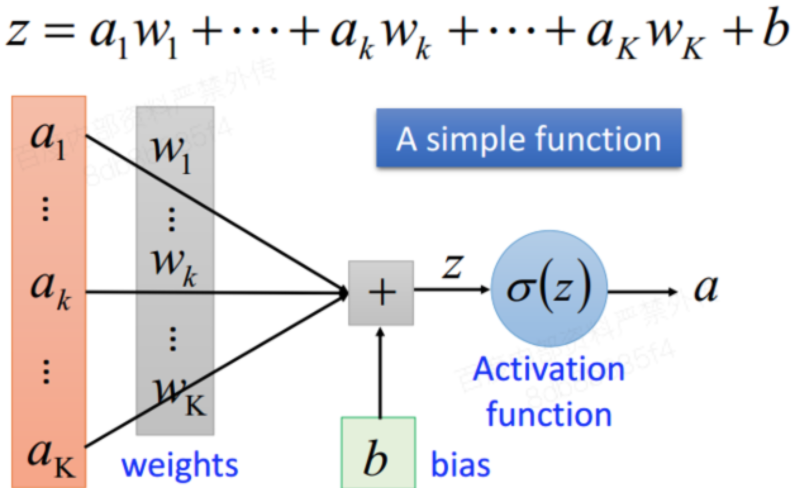# 教程2：一个深度学习的典型场景

## 波士顿房价预测问题

### 2 教程

#### 2.1 启动notebook编写代码打开Notebook，新建1个notebook文件：house_pre.ipynb

首先导入必要的包，分别是：

numpy--------->python基本库，用于科学计算

os--------------->python的模块，可使用该模块对操作系统进行操作

matplotlib----->python绘图库，可方便绘制折线图、散点图等图形

``````import paddle.fluid as fluid
import numpy as np
import os
import matplotlib.pyplot as plt``````

#### Step1：准备数据

波士顿房价数据集介绍： 该数据集共506行,每行14列。前13列用来描述房屋的各种属性（如城镇人均犯罪率等），最后一列为该类房屋价格中位数。波士顿房价数据集的属性标签及其含义如下：

属性 含义 属性 含义 属性 含义
CRIM 城镇人均犯罪率 LSTAT 地区中有多少房东属于低收入人群 TAX 每一万美元的不动产税率
RM 每栋住宅的房间数 AGE 1940 年以前建成的自住单位的比例 PTRATIO 城镇中的教师学生比例
ZN 住宅用地所占比例 DIS 距离 5 个波士顿的就业中心的加权距离 RAD 距离高速公路的便利指数
B 城镇中的黑人比例 INDUS 城镇中非住宅用地所占比例 CHAS 虚拟变量,用于回归分析
NOX 环保指数 MEDV 自住房屋房价中位数（也就是均价）

``````BUF_SIZE=500
BATCH_SIZE=20

#用于训练的数据提供器，每次从缓存中随机读取批次大小的数据
buf_size=BUF_SIZE),
batch_size=BATCH_SIZE)
#用于测试的数据提供器，每次从缓存中随机读取批次大小的数据
buf_size=BUF_SIZE),
batch_size=BATCH_SIZE)  ``````

``````#用于打印，查看uci_housing数据
sampledata=next(train_data())
print(sampledata)``````

打印结果如下图所示：#### Step2：网络配置

（1）网络搭建

对于线性回归来讲，它就是一个从输入到输出的简单的全连接层。

对于波士顿房价数据集，假设属性和房价之间的关系可以被属性间的线性组合描述。``````#定义张量变量x，表示13维的特征值
x = fluid.layers.data(name='x', shape=, dtype='float32')
#定义张量y,表示目标值
y = fluid.layers.data(name='y', shape=, dtype='float32')
#定义一个简单的线性网络,连接输入和输出的全连接层
#input:输入tensor;
#size:该层输出单元的数目
#act:激活函数
y_predict=fluid.layers.fc(input=x,size=1,act=None)``````

(2)定义损失函数

此处使用均方差损失函数。

square_error_cost(input,lable):接受输入预测值和目标值，并返回方差估计,即为（y-y_predict）的平方。

``````cost = fluid.layers.square_error_cost(input=y_predict, label=y) #求一个batch的损失值
avg_cost = fluid.layers.mean(cost)                              #对损失值求平均值``````

(3)定义优化函数

此处使用的是随机梯度下降。

``````optimizer = fluid.optimizer.SGDOptimizer(learning_rate=0.001)
opts = optimizer.minimize(avg_cost)``````

在上述模型配置完毕后，得到两个fluid.Program：fluid.default_startup_program() 与fluid.default_main_program() 配置完毕了。

参数初始化操作会被写入fluid.default_startup_program()。

fluid.default_main_program()用于获取默认或全局main program(主程序)。该主程序用于训练和测试模型。fluid.layers 中的所有layer函数可以向 default_main_program 中添加算子和变量。default_main_program 是fluid的许多编程接口（API）的Program参数的缺省值。例如,当用户program没有传入的时候， Executor.run() 会默认执行 default_main_program 。

``test_program = fluid.default_main_program().clone(for_test=True)``

#### Step3：模型训练 & Step4：模型评估

（1）创建Executor

首先定义运算场所 fluid.CPUPlace()和 fluid.CUDAPlace(0)分别表示运算场所为CPU和GPU。

Executor:接收传入的program，通过run()方法运行program。

``````use_cuda = False                         #use_cuda为False,表示运算场所为CPU;use_cuda为True,表示运算场所为GPU
place = fluid.CUDAPlace(0) if use_cuda else fluid.CPUPlace()
exe = fluid.Executor(place)              #创建一个Executor实例exe
exe.run(fluid.default_startup_program()) #Executor的run()方法执行startup_program(),进行参数初始化``````

（2）定义输入数据维度

feed_list设置向模型输入的向变量表或者变量表名

``````# 定义输入数据维度
feeder = fluid.DataFeeder(place=place, feed_list=[x, y])#feed_list:向模型输入的变量表或变量表名``````

（3）定义绘制训练过程的损失值变化趋势的方法draw_train_process

``````iter=0;
iters=[ ]
train_costs=[ ]

def draw_train_process(iters,train_costs):
title="training cost"
plt.title(title, fontsize=24)
plt.xlabel("iter", fontsize=14)
plt.ylabel("cost", fontsize=14)
plt.plot(iters, train_costs,color='red',label='training cost')
plt.grid()
plt.show()``````

（4）训练并保存模型

Executor接收传入的program,并根据feed map(输入映射表)和fetch_list(结果获取表) 向program中添加feed operators(数据输入算子)和fetch operators（结果获取算子)。 feed map为该program提供输入数据。fetch_list提供program训练结束后用户预期的变量。enumerate() 函数用于将一个可遍历的数据对象(如列表、元组或字符串)组合为一个索引序列，同时列出数据和数据下标。

``````EPOCH_NUM=50

model_save_dir = "./fit_a_line.inference.model" #本地任务模型保存地址

for pass_id in range(EPOCH_NUM):                                  #训练EPOCH_NUM轮
# 开始训练并输出最后一个batch的损失值
train_cost = 0
train_cost = exe.run(program=fluid.default_main_program(),#运行主程序
feed=feeder.feed(data),              #喂入一个batch的训练数据，根据feed_list和data提供的信息，将输入数据转成一种特殊的数据结构
fetch_list=[avg_cost])
if batch_id % 40 == 0:
print("Pass:%d, Cost:%0.5f" % (pass_id, train_cost))    #打印最后一个batch的损失值
iter=iter+BATCH_SIZE
iters.append(iter)
train_costs.append(train_cost)

# 开始测试并输出最后一个batch的损失值
test_cost = 0
test_cost= exe.run(program=test_program, #运行测试cheng
feed=feeder.feed(data),               #喂入一个batch的测试数据
fetch_list=[avg_cost])                #fetch均方误差
print('Test:%d, Cost:%0.5f' % (pass_id, test_cost))     #打印最后一个batch的损失值

#保存模型
# 如果保存路径不存在就创建
if not os.path.exists(model_save_dir):
os.makedirs(model_save_dir)
print ('save models to %s' % (model_save_dir))
#保存训练参数到指定路径中，构建一个专门用预测的program
fluid.io.save_inference_model(model_save_dir,   #保存推理model的路径
['x'],            #推理（inference）需要 feed 的数据
[y_predict],      #保存推理（inference）结果的 Variables
exe)              #exe 保存 inference model
draw_train_process(iters,train_costs)``````

注：提交云端任务时，模型保存地址改为：

``````output_path = os.environ['OUTPUT_PATH']  #输出路径
model_save_dir = os.path.join(output_path, 'fit_a_line.inference.model') #模型保存路径``````

运行代码，打印训练和测试的损失：#### Step5：模型预测

（1）创建预测用的Executor

``````infer_exe = fluid.Executor(place)    #创建推测用的executor
inference_scope = fluid.core.Scope() #Scope指定作用域``````

（2）可视化真实值与预测值方法定义

``````infer_results=[ ]
groud_truths=[ ]

#绘制真实值和预测值对比图
def draw_infer_result(groud_truths,infer_results):
title='Boston'
plt.title(title, fontsize=24)
x = np.arange(1,20)
y = x
plt.plot(x, y)
plt.xlabel('ground truth', fontsize=14)
plt.ylabel('infer result', fontsize=14)
plt.scatter(groud_truths, infer_results,color='green',label='training cost')
plt.grid()
plt.show()``````

(3) 开始预测

``````with fluid.scope_guard(inference_scope):#修改全局/默认作用域（scope）, 运行时中的所有变量都将分配给新的scope。
#从指定目录中加载 推理model(inference model)
[inference_program,                             #推理的program
feed_target_names,                             #需要在推理program中提供数据的变量名称
model_save_dir, #model_save_dir:模型训练路径
infer_exe)      #infer_exe: 预测用executor
#获取预测数据
batch_size=200)                           #从测试数据中读取一个大小为200的batch数据
test_x = np.array([data for data in test_data]).astype("float32")
test_y= np.array([data for data in test_data]).astype("float32")
results = infer_exe.run(inference_program,                              #预测模型
feed={feed_target_names: np.array(test_x)},  #喂入要预测的x值
fetch_list=fetch_targets)                       #得到推测结果

print("infer results: (House Price)")
for idx, val in enumerate(results):
print("%d: %.2f" % (idx, val))
infer_results.append(val)
print("ground truth:")
for idx, val in enumerate(test_y):
print("%d: %.2f" % (idx, val))
groud_truths.append(val)
draw_infer_result(groud_truths,infer_results)``````

运行代码，输出房价的预测值：### 2.2 提交本地/云端任务

编写完代码后，返回BML CodeLab桌面进入"任务"应用。#### Step1：创建任务

填写任务名称，选择相应的执行方式和启动方式，提交本地任务，详见本地任务管理(https://cloud.baidu.com/doc/BML/s/Lkgbzlof8)。#### Step2：查看运行结果

点击"提交"任务后，在本地任务查看训练和评估结果。#### 2.2.2 提交云端任务

提交云端任务也需要"创建任务"、"查看运行结果"，详见云端任务管理(https://cloud.baidu.com/doc/BML/s/3kgbzmmos)。与提交本地任务不同的是：

1. 提交云端任务前，需要先把notebook文件上传到云端。

点击"本地推送到云端"按钮。选择需要上传的文件：house_pre.ipynb，点击"提交"。弹窗中将显示修改的文件，不同文件颜色代表不同含义，如标绿文件为新建文件，红色文件为已删除文件，蓝色文件为已修改文件。点击"历史版本"。查看新生成的版本:如V17。点击某一版本号将显示当前版本的详细信息。如果未将文件上传至云端：

• 首次创建任务时,云端文件版本将为空，无法创建云端任务；
• 非首次创建任务时，无法选择含有指定文件的云端文件版本，创建任务后，任务将运行失败。2. 提交云端任务时，为保证不同任务之间的环境不会相互影响，云端会对每个任务的产出做隔离。云端在执行任务前会自动注入环境变量 `OUTPUT_PATH` , 用户在训练过程中产生的数据，模型、运行结果均可写入到此环境变量对应的地址中， 任务执行完成后，该地址中的数据将会自动上传。用户即可在本地浏览任务产生的数据。

提交本地任务时，运行结果可以保存到任意位置。

用法示例：

``````# 云端数据输出地址，任务执行完成后，该地址下面的所有数据/代码将会被上传
output_path = os.environ['OUTPUT_PATH']  #输出路径
model_path = os.path.join(output_path, 'fit_a_line_model') #模型保存路径
train_data_path = os.path.join(output_path, 'train_data')#训练数据保存路径
test_data_path = os.path.join(output_path, 'test_data')#测试数据保存路径

with open(train_data_path, 'w') as fwriter:
fwriter.write("train data")

with open(test_data_path, 'w') as fwriter:
fwriter.write("test data")``````